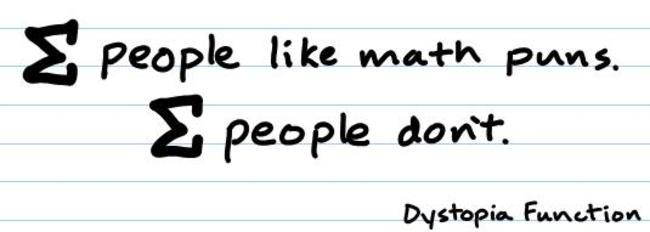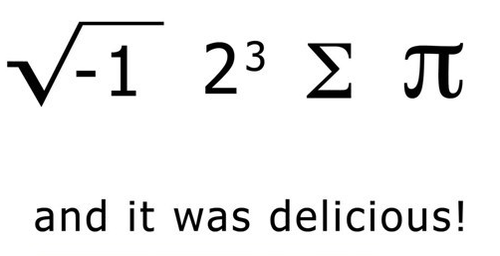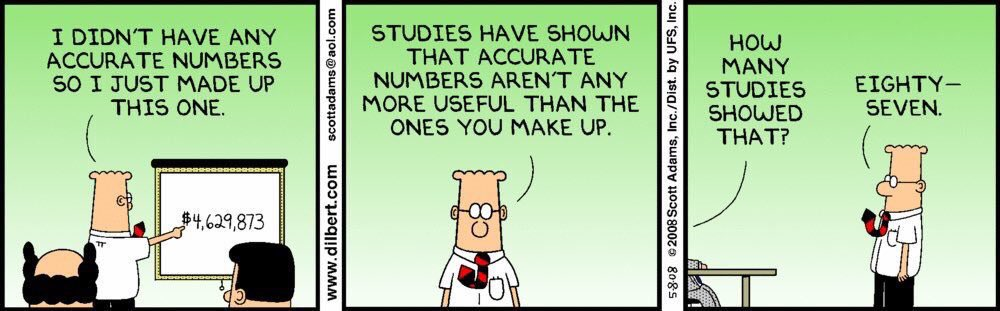## Humour (154)

28 février 2019Publicités

22 février 2017

## Pythagorean Theorem

20 novembre 2016That’s the simplest proof of the Pythagorean Theorem, here.

And if you’re really curious, there’s plenty of other ways to prove the theorem!

## Humour (98)

8 août 2016## Math blogs

7 août 2016Looking for some cool math blogs?  Here are some I like…

English

French

## Humour (91)

3 août 2016## Cryptarithm

1 août 2016

It all started this morning with a very short post in the newsgroup rec.puzzles :

This is a cryptarithm.

TRUTH * IS = WISDOM

Each letter is a different digit; T appears twice and represents the same digit, and so on; base 10.

I’ve solved puzzles similar to this one in the past but usually, I had a few hints or givens and it involved addition, not multiplication!  So this one seemed more complex and interesting!

The method for solving this kind of puzzle is more or less always the same : convert arithmetic operations into algebra (or sets of possible values), eliminate a few candidate values by deduction, do some simple substitutions in the equations you get and voilà!  But this time, I had to work a lot harder!

Let’s start with a graphic that details what goes on when multiplying the number TRUTH by the number IS to get the product, the number WISDOM.

The trick here is to list everything we know (or can deduct) about this product.

From the S * TRUTH

1) (S * H) = a + (10 * b)
2) (S * T) + b = c + (10 * d)
3) (S * U) + d = e + (10 * f)
4) (S * R) + f = g + (10 * h)
5) (S * T) + h = i + (10 * j)

From I * TRUTH
6) (I * H) = k + (10 * l)
7) (I * T) + l = m + (10 * n)
8) (I * U) + n = o + (10 * p)
9) (I * R) + p = q + (10 * r)
10) (I * T) + r = s + (10 * t)

From the intermediate results of Line 3 + Line 4
11) a + 0 = M + (10 * u), this is a + ZERO = M + (10 * u)
12) u + c + k = O + (10 * v)
13) v + e + m = D + (10 * w)
14) w + g + o = S + (10 * x)
15) x + i + q = I + (10 * y)
16) y + s + j + t = W

From the numbers themselves

We know that the 3 numbers cannot start with the digit zero so :
17) 1 <= W <= 9, or W <> 0
18) 1 <= I <= 9, or I <> 0
19) 1 <= T <= 9, or T <> 0

Since TRUTH is a 5-digit number, it has to be less than 99999.  To be more precise, since all letter-digits have to be different, TRUTH would have to be less than the maximum possible (98765) but, because of the repeating digit T, we have:

20) 10000T + 1000R + 100U + 10T + H <= 98796

Same reasoning for IS :

21) (10*I) + S <= 98

Finally, for WISDOM
22) 100000W + (10000*I) + 1000S + 100D + (10*O) + D <= 987654

Same logic as above can now be applied to minimum values :

23) 10000T + 1000R + 100U + 10T + H >= 10213
24) (10*I) + S >= 10
25) 100000W + (10000*I) + 1000S + 100D + (10*O) + M >= 102345

One remark about H and S :  if H or S would be zero, the resulting digit from this product, M, would also be zero.  That’s a contradiction since all digits have to be different, therefore,

26) 1 <= H <= 9, or H <> 0
27) 1 <= S <= 9, or S <> 0

From the carry

Now, let’s have a look at the possible values for every carry.  Let’s start with an example

Since all digits have to be different, the maximum result we can have from a multiplication in our case is 9×8 = 8×9 = 72, therefore the maximum value for all carry variables is 7.  So,

28) b <= 7
29) d <= 7
30) f <= 7
31) h <= 7
32) j <= 7
33) l <= 7
34) n <= 7
35) p <= 7
36) r <= 7
37) t <= 7

For the addition carry (Carry of line 3 + line 4), since they only involve 2 digits, the maximum is 9+8 = 8+9 = 17, therefore the carry must be zero or one.  So,
38) 0 <= u <= 1
39) 0 <= v <= 1
40) 0 <= w <= 1
41) 0 <= x <= 1
42) 0 <= y <= 1

Now, you can throw in a few modular equations and arithmetic, build sets of possible values and carefully examine W and use the fact that the multiplication I*T appears twice.  Also take advantage of the fact that all equations are diophantine equations! It’s also very useful to notice the fact that T, H, S, I and W cannot be zero! I hope you have enough to get you started!

I hope you didn’t think I’d spoil all the fun, don’t you?  The rest is left as an exercise to the reader as they say!

Oh, by the way, I verified and there’s only one solution!  ;)